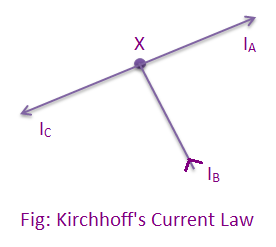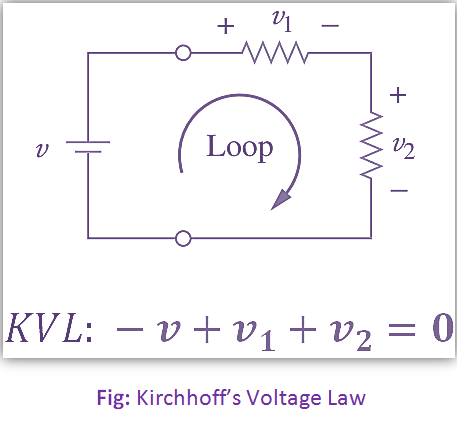# Kirchhoff’s Law Derivation

When the electrical networks can’t be solved easily by ohm’s law, then we take help from different laws and theorems to save time and reduce mathematical calculations. So, we take the help of the following law and theorem:

## Kirchhoff’s Law:

Kirchhoff’s laws comprises of two laws:

i. Current or Point Law: The algebraic sum of the current meeting at any point is zero in any electric network. This law proves that
incoming current = outgoing currentii. Voltage or Mesh Law: This law states that in any closed mesh the algebraic sum of the voltage drops in each conductor plus the algebraic sum of e.m.f’s is zero. Therefore, in a closed mesh
∴ ∑IR+ ∑E = 0